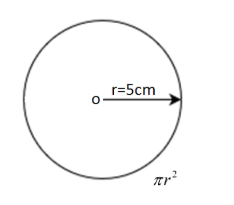Find the area of a circle when radius

# Find the area of a circle when radius1. A2. B3. C4. D
None of these

Fill Out the Form for Expert Academic Guidance!l

+91

Live ClassesBooksTest SeriesSelf Learning

Verify OTP Code (required)

### Solution:

Concept- The question asks you to find the area of ​​a circle with a given radius. To do this, we need to know the formula for the circle, that is,. The formula is simple and you need the radius of the circle to find its area.From the question we know it –Here, we assign the radius value to the square area formula.The area of ​​a circle isHence, option 1 is correct.

## Related content

 Area of Square Area of Isosceles Triangle Pythagoras Theorem Triangle Formula Perimeter of Triangle Formula Area Formulae Volume of Cone Formula Matrices and Determinants_mathematics Critical Points Solved Examples Type of relations_mathematics+91

Live ClassesBooksTest SeriesSelf Learning

Verify OTP Code (required)